# TSA Entropy VI

LabVIEW 2014 Advanced Signal Processing Toolkit Help

Edition Date: June 2014

Part Number: 372656C-01

»View Product InfoDownload Help (Windows Only)

Owning Palette: Statistical Analysis VIs

Computes the entropy of a univariate or multivariate (vector) time series. Wire data to the Xt input to determine the polymorphic instance to use or manually select the instance.

Use the pull-down menu to select an instance of this VI.

 Select an instance TSA Entropy (Waveform)TSA Entropy (Array)TSA Vector Entropy (Waveform)TSA Vector Entropy (Array)

## TSA Entropy (Waveform)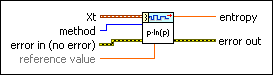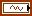Xt specifies the univariate time series.method specifies the method to use in computing the entropy of the time series. Options include Shannon (default), Threshold, Sure, Norm, and Energy. Refer to the Details section for more information about each method.error in describes error conditions that occur before this node runs. This input provides standard error in functionality.reference value specifies the associated parameter for the methods Threshold, Sure, and Norm. For the Threshold and Sure methods, the reference value must be greater than 0. For the Norm method, the reference value must be greater than 1.entropy returns the entropy of the time series.error out contains error information. This output provides standard error out functionality.

## TSA Entropy (Array)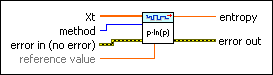Xt specifies the univariate time series.method specifies the method to use in computing the entropy of the time series. Options include Shannon (default), Threshold, Sure, Norm, and Energy. Refer to the Details section for more information about each method.error in describes error conditions that occur before this node runs. This input provides standard error in functionality.reference value specifies the associated parameter for the methods Threshold, Sure, and Norm. For the Threshold and Sure methods, the reference value must be greater than 0. For the Norm method, the reference value must be greater than 1.entropy returns the entropy of the time series.error out contains error information. This output provides standard error out functionality.

## TSA Vector Entropy (Waveform)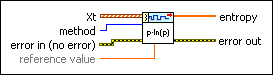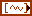Xt specifies the multivariate (vector) time series.method specifies the method to use in computing the entropy of the time series. Options include Shannon (default), Threshold, Sure, Norm, and Energy. Refer to the Details section for more information about each method.error in describes error conditions that occur before this node runs. This input provides standard error in functionality.reference value specifies the associated parameter for the methods Threshold, Sure, and Norm. For the Threshold and Sure methods, the reference value must be greater than 0. For the Norm method, the reference value must be greater than 1.entropy returns the vector entropy of the multivariate (vector) time series.error out contains error information. This output provides standard error out functionality.

## TSA Vector Entropy (Array)Xt specifies the multivariate (vector) time series. Each column of the 2D array represents a vector at certain time.method specifies the method to use in computing the entropy of the time series. Options include Shannon (default), Threshold, Sure, Norm, and Energy. Refer to the Details section for more information about each method.error in describes error conditions that occur before this node runs. This input provides standard error in functionality.reference value specifies the associated parameter for the methods Threshold, Sure, and Norm. For the Threshold and Sure methods, the reference value must be greater than 0. For the Norm method, the reference value must be greater than 1.entropy returns the vector entropy of the multivariate (vector) time series.error out contains error information. This output provides standard error out functionality.

## TSA Entropy Details

This VI computes the Shannon entropy according to the following equation: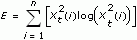When Xt2(i)=0, let log(Xt2(i))=0. n is the number of samples of the input time series.

This VI computes the Threshold entropy according to the following equation: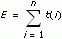where t(i)=1 if abs(Xt(i))>p and 0 elsewhere. n is the number of samples of the input time series, and p is reference value.

This VI computes the Sure entropy according to the following equation: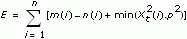where m(i)=1 if abs(Xt(i))>p and 0 elsewhere, n(i)=1 if abs(Xt(i))≥p and 0 elsewhere

n is number of samples of the input time series, and p is reference value.

This VI computes the Norm entropy according to the following equation: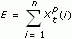where n is the number of samples of the input time series. p is reference value.

This VI computes the Energy entropy according to the following equation: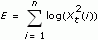When Xt2(i)=0, let log(Xt2(i))=0. n is the number of samples of the input time series.

## Example

Refer to the Series Statistical Analysis VI in the labview\examples\Time Series Analysis\TSAGettingStarted directory for an example of using the TSA Entropy VI.